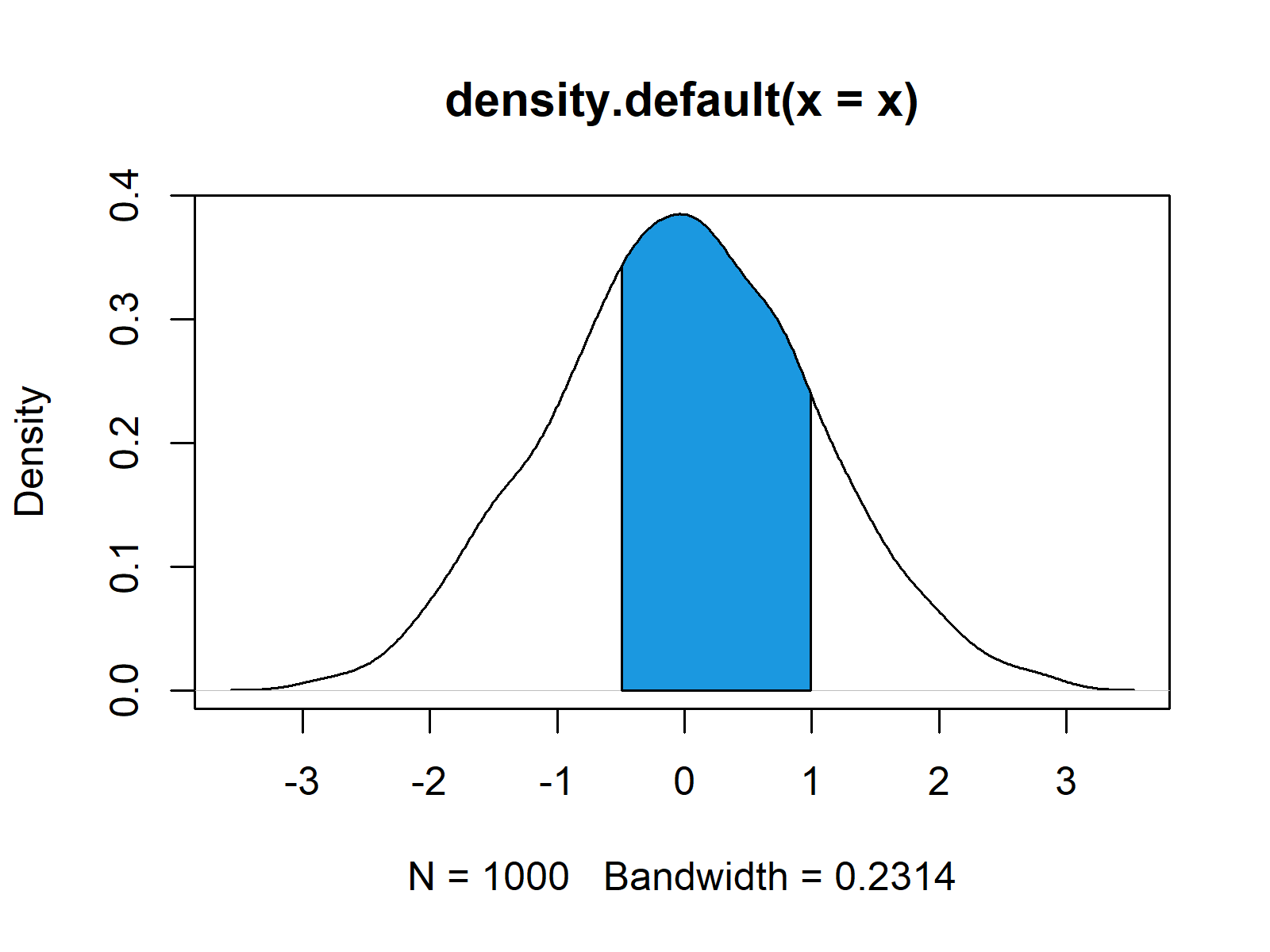# Add Color Between Two Points of Kernel Density Plot in R (Example)

In this tutorial, I’ll show how to add color to a certain area below a density plot in the R programming language.

The content of the post looks as follows:

You’re here for the answer, so let’s get straight to the examples!

## Introducing Example Data

First, we need to generate some random data that we can draw as kernel density plot later on:

```set.seed(75757)                                            # Create example data
x <- rnorm(1000)```

Our example data consists of 1000 numeric values and is normally distributed.

Now, we can convert our numeric vector to a density object with the density function:

`dens_x <- density(x)                                       # Compute density of data`

We can draw a graph with default specifications by using the plot function:

`plot(dens_x)                                               # Default plot of density`Figure 1: Basic Density Plot in R.

Figure 1 shows how our example plot looks like. It’s a normally distributed kernel density graph with a mean of 0 and a standard deviation of 1.

## Example: Coloring Particular Area Below Density Plot

If we want to add some color or a shade below our kernel density plot, we first need to specify between which points on the x-axis we want to add this color. In this example, we are using the lower limit – 0.5 and the upper limit 1:

```x_low <- min(which(dens_x\$x >= - 0.5))                     # Define lower limit of colored area
x_high <- max(which(dens_x\$x < 1))                         # Define upper limit of colored area```

Now, we can use the with and the polygon functions to add some color between our two limits:

```with(dens_x,                                               # Add color between two limits
polygon(x = c(x[c(x_low, x_low:x_high, x_high)]),
y = c(0, y[x_low:x_high], 0),
col = "#1b98e0"))```Figure 2: Density with Colored Area Between Two Points.

Figure 2 illustrates the output of the previous R programming code. As you can see, we added some blue color below the previously specified area of our graphic.

## Video & Further Resources

Do you want to learn more about graphics in R? Then you might want to watch the following video which I have published on my YouTube channel. In the video, I show the R programming code of this page.

Please accept YouTube cookies to play this video. By accepting you will be accessing content from YouTube, a service provided by an external third party.If you accept this notice, your choice will be saved and the page will refresh.

Furthermore, you may have a look at this forum thread at Stack Overflow. The R code shown in this tread was used as basement for this tutorial and in the thread you can also find R programming codes for the coloring of certain areas below a density based on the ggplot2 package.

In addition, you may want to have a look at some of the related articles on my website. You can find a selection of articles below:

Summary: You learned in this tutorial how to shade a particular part of a kernel density graphic in the R programming language. Let me know in the comments, if you have any additional questions.

Subscribe to the Statistics Globe Newsletter